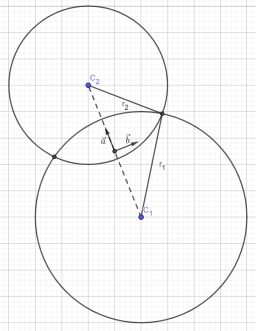# Complex equation

If c - d = 2 and c = 12 + 7 i, find d. Write the result in the form of a + bi.

d = 10+7i

### Step-by-step explanation:Did you find an error or inaccuracy? Feel free to write us. Thank you!

Tips for related online calculators
Do you have a linear equation or system of equations and looking for its solution? Or do you have a quadratic equation?
Try our complex numbers calculator.